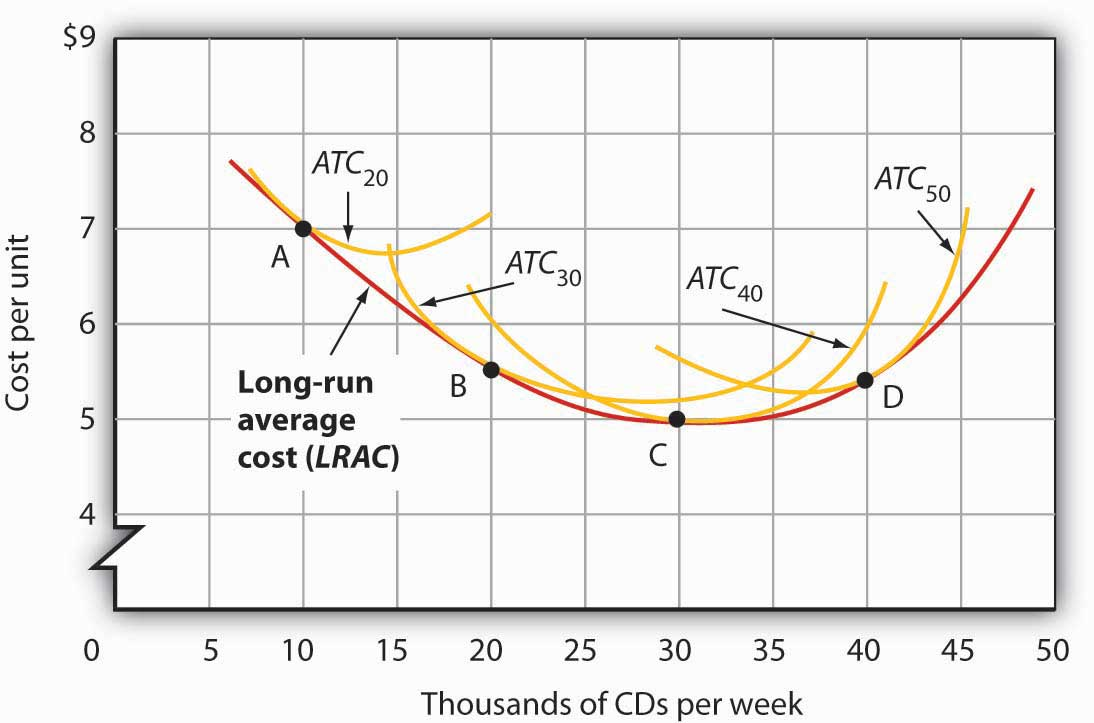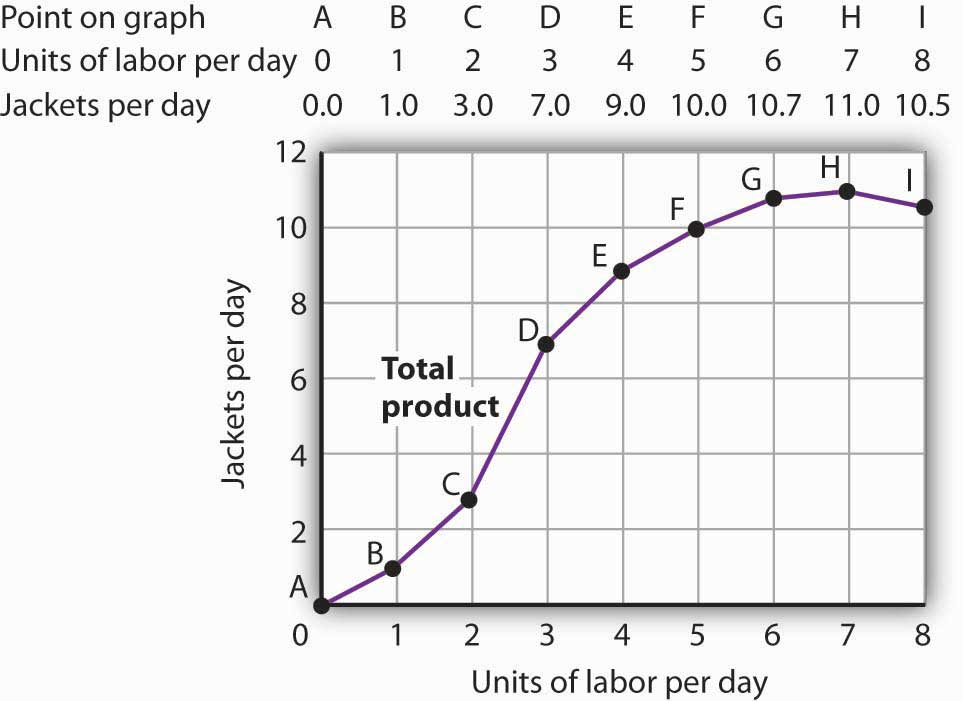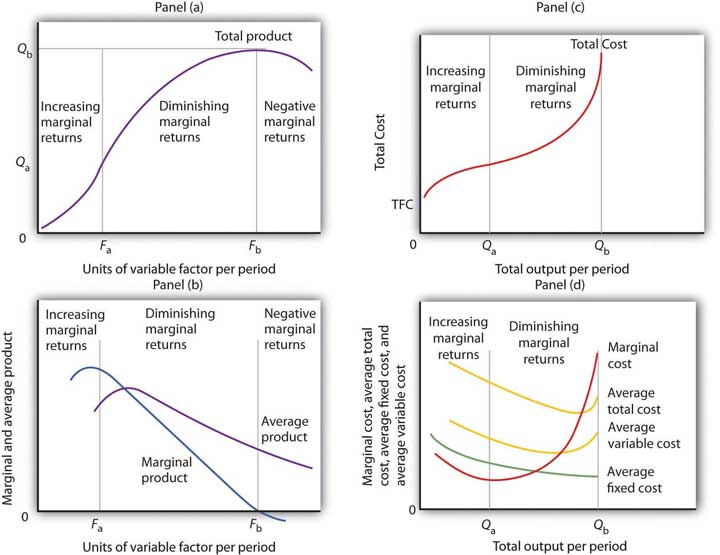## Unit 5 Study Guide: The Producer

#### 5a: Analyze the behavior of the producer.

• Define and explain the difference between accounting profit and economic profit.
• Define opportunity costs and explain how they affect a company's production decisions?

In this unit we analyze how businesses or producers make decisions. For example, businesses decide how much they should produce of the item they are selling, the price they should charge customers, and the number of hours they should operate. Costs and revenues are important considerations for companies that aim to maximize profit.

It is important to distinguish between accounting profit and economic profit. Companies make decisions based on their goal to maximize economic, not accounting, profit. For example, a company may be able to earn a positive accounting profit, but determine it is best to close its operations if economic profit remains negative.

Economic profits offer businesses a useful tool for making decisions. For example, consider an individual who quits their corporate job (earning $60,000 a year) to start a small business that earns$40,000 a year in accounting profits. The positive accounting profit indicates the business is able to cover its costs and earn positive profits. However, when the business owner considers economic profit of -$20,000 (calculated as accounting profit minus the opportunity cost of the forgone salary) they determine it may be best to close the business and return to earning their previous corporate salary. Review the decision making process companies face in the following resources. #### 5b: Compare the costs of production in the short run to costs of production in the long run. • Define a short-run costlong-run cost, and respective cost. • Define and name some examples of fixed costsvariable costs and total costs. • Define average total cost and explain how to compute it. • Define marginal cost and explain how to compute it. • How do costs change when we increase or decrease output? Economics define profits as the total revenues minus the total costs (which include opportunity costs). While revenues largely depend on the demand and consumer behavior, businesses have direct control over their costs. In this section we analyze a company's cost structure and its effect on profitability and production decisions. Review how to distinguish between short-run and long-run costs, since they affect outcomes in different ways. Short-Run Costs The short run describes a time period when many variables are fixed. In the long run, all variables are flexible. Consider an ice cream shop that leases an ice cream machine and has contracted two employees to work on an hourly basis (at$10 per hour). The cost to rent the storefront and the cost to lease the ice cream machine are fixed: the ice cream shop must pay these fixed costs regardless of how many ice cream cones it produces or sells. How do fixed costs behave when we increase the number of ice cream cones produced?

Since the business manager has contracted its employees to work on an hourly basis, the number of work hours will vary with the level of production (number of ice cream cones produced and sold). This cost is variable not fixed. We can see in the table that larger quantities of production are associated with more hours worked.

Ice Cream Shop Costs

 Ice CreamCones Fixed Cost Variable Cost Total Cost Average TotalCost Marginal Cost 0 $1,000$0 $1,000 - - 1$1,000 $10$1,010 $1,010$10 5 $1,000$10 $1,010$202 $0 10$1,000 $30$1,030 $103$20 15 $1,000$60 $1,060$71 $30 20$1,000 $100$1,100 $55$40

Review the theory of costs and some visual examples in Production Choices and Costs: The Short Run from Principles of Microeconomics.

• How can we interpret the region of the long-run average cost (LRAC) where costs are falling (points A and B)?
• How can we interpret the region of the long-run average cost (LRAC) where costs are rising (point D)?
• What is the meaning of the lowest point on the long-run average cost (LRAC) curve (point C)?

Long-Run Costs

The long run describes a time period in which all costs are variable.

For example, a production facility or factory may be a fixed variable in the short run, but with sufficient time in the long run, the business owner could build a new facility in a new location, close an existing operation, expand or reduce its production capacity. The factory itself becomes a variable resources in the long run.

For example, consider Figure 8.9, which illustrates the long-run cost curve of a company producing CDs. Note that the long-run average total cost curve envelopes its short-run cost average total cost curves.

Figure 8.9 Relationship between Short-Run and Long-Run Average Total CostsWe derive the long-run average cost (LRAC) by taking the lowest average total cost curve at each level of output. The graph displays the average total cost curves for 20, 30, 40, and 50 units of capital (the four smaller yellow curves).

• When the CD company produces 10,000 CDs per week, it can minimize the cost per CD by producing with 20 units of capital (point A).
• When the CD company produces 20,000 CDs per week, with an expansion in plant size, it can minimize the cost per CD by producing with 30 units of capital (point B).
• The CD company achieves the lowest cost per unit when it produces 30,000 CDs per week using 40 units of capital (point C).
• If the CD company decides to produce 40,000 CDs per week, it will do so most cheaply with 50 units of capital (point D).
Review long-run costs in the following resources.

#### 5c: Identify the production function in the short run as well as the long run.

• What are the goals of the company when choosing a specific combination of inputs and a production function?

The Production Function

A production function is the relationship between a company's factors of production and its output. For example, the ice cream shop from the previous section uses inputs: fixed resources (the shop and the ice cream machine) and variable resources (employees, sugar, cream, cones) to produce output: ice cream cones.

• What could cause an increase in labor productivity? Think about specialization and trade.

As we examine the company's production choices, we consider factors that contribute to its profitability, such as the productivity of its resources.

Figure 8.1 presents an example of a company that produces jackets. The table above the graph indicates output levels per day for Acme Clothing Company at various quantities of labor per day, assuming the company's capital is fixed.

Figure 8.1 Acme Clothing's Total Product Curve

####We plot these values on the graph as a total product curve. As we move from points A, B, C to D, the slope of the production function rises, indicating increased worker productivity.

• What could cause a decrease in labor productivity? Think about the effect of crowding out a small space with too many employees.

However, as we continue adding more employees past point D, labor productivity declines (diminishing marginal product) until it reaches point H. After point H, further additions of labor will actually lead to negative returns in production.

• Define the law of diminishing marginal returns.

This type of outcome in production is so common, that economists define it as the law of diminishing marginal returns, which holds that the marginal product of any variable factor of production will eventually decline, assuming the quantities of other factors of production are unchanged.

Review the production function in the following resources.

• Define the company shut down decision.
• How do its average total cost and average variable cost influence this decision in the short and long runs?

The Company Shut-Down Decision

Have you been to a store or restaurant when you were the only customer? You may have wondered why the owner chose to keep the restaurant open at this time of the day or week. However, this decision to remain open during low customer traffic times may be a profit maximizing (or loss minimizing) choice.

The shutdown rule refers to the times when a company chooses to remain open during times of low activity, provided it can cover its variable costs with any revenue it generates. The company weighs whether it is better to incur a certain loss by staying open, or incur an even bigger loss by closing during these times. Remember, it must pay its fixed costs regardless of whether it stays open or closes.

Review the shut-down rule in this video Understanding the Short-Run Shutdown.

#### 5d: Compute the relationship between different cost functions.

• What specific calculations define each type of cost?
• What does a graph of the respective cost curve look like?
• How does the slope of each graph change in response to changes in output?

Make sure you can define the following cost functions, describe how each variable affects production choices, draw each cost curve on a graph, and analyze their shapes and interactions.

• Fixed costs;
• Variable costs;
• Total costs;
• Marginal costs;
• Average fixed costs;
• Average variable costs; and
• Average total costs.

For our ice cream shop example, consider how fixed costs and average fixed costs behave as the shop produces more ice cream cones. Remember that fixed costs will remain the same regardless of how many ice cream cones are produced. Therefore, the average fixed cost must decline with each additional unit produced. The shop will lower per unit costs of production by raising output.

With respect to variable costs, note that average variable costs increase as production increases. What will that look like on a graph? Consider the effect on worker productivity, as you add additional employees in a crowded space and as you ask employees to work extra long hours.

How do you calculate marginal cost from total cost and what is the interpretation of an increasing marginal cost? Again, consider the effect on worker productivity, as you add additional employees in a crowded space and as you ask employees to work extra long hours.

• Why is the average fixed cost a downward sloping function?
• Why are the marginal cost, average variable cost, and average total cost U-shaped?
• Why does the marginal cost curve cross the average total cost and the average variable cost curve exactly at their minimum points?

Ice Cream Shop Costs

 Ice CreamCones Fixed Cost Average FixedCost Variable Cost Average VariableCost Total Cost Average TotalCost Marginal Cost 0 $1,000 -$0 - $1,000 - - 1$1,000 $200$10 $10$1,010 $1,010$10 5 $1,000$200 $10$2 $1,010$202 $0 10$1,000 $100$30 $3$1,030 $103$20 15 $1,000$67 $60$4 $1,060$71 $30 20$1,000 $50$100 $5$1,100 $55$40

Once you master the definitions, you can put these costs together on the same graph and examine their interactions and dependencies.

Key Takeaways from 8.1 Production Choices and Costs: The Short Run

####In Panel (a), the total product curve for a variable factor in the short run shows that the firm experiences increasing marginal returns from zero to Fa units of the variable factor (zero to Qa units of output), diminishing marginal returns from Fa to Fb (Qa to Qb units of output), and negative marginal returns beyond Fb units of the variable factor.

Panel (b) shows that marginal product rises over the range of increasing marginal returns, falls over the range of diminishing marginal returns, and becomes negative over the range of negative marginal returns. Average product rises when marginal product is above it and falls when marginal product is below it.

In Panel (c), total cost rises at a decreasing rate over the range of output from zero to Qa. This was the range of output that was shown in Panel (a) to exhibit increasing marginal returns. Beyond Qa, the range of diminishing marginal returns, total cost rises at an increasing rate. The total cost at zero units of output (shown as the intercept on the vertical axis) is total fixed cost.

Panel (d) shows that marginal cost falls over the range of increasing marginal returns, then rises over the range of diminishing marginal returns. The marginal cost curve intersects the average total cost and average variable cost curves at their lowest points. Average fixed cost falls as output increases. Note that average total cost equals average variable cost plus average fixed cost.

Review this material in the following resources.

#### Unit 5 Vocabulary

• Accounting profit
• Average fixed costs
• Average product
• Average total costs
• Average variable costs
• Company shutdown rule
• Cost function
• Constant returns to scale
• Diminishing marginal product
• Diseconomies of scale
• Economic profit
• Economies of scale
• Fixed costs
• Labor productivity
• Law of diminishing marginal returns
• Long-run cost
• Long-run average cost
• Marginal cost
• Marginal product
• Marginal product of labor
• Opportunity cost
• Production function
• Respective cost
• Short-run cost
• Short-run average cost
• Total costs
• Variable costs